# Maharashtra Board Practice Set 16 Class 6 Maths Solutions Chapter 5 Decimal Fractions

Balbharti Maharashtra State Board Class 6 Maths Solutions covers the Std 6 Maths Chapter 5 Decimal Fractions Class 6 Practice Set 16 Answers Solutions.

## 6th Standard Maths Practice Set 16 Answers Chapter 5 Decimal Fractions

Question 1.
If, 317 × 45 = 14265, then 3.17 × 4.5 = ?
Solution:
3.17 × 4.5
= 14.265

Question 2.
If, 503 × 217 = 109151, then 5.03 × 2.17 = ?
Solution:
5.03 x 2.17
= 10.9151

Question 3.
i. 2.7 × 1.4
ii. 6.17 × 3.9
iii. 0.57 × 2
iv. 5.04 × 0.7
Solution:
i. 2.7 × 1.4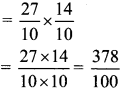= 3.75

ii. 6.17 × 3.9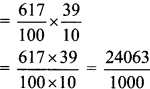= 24.063

iii. 0.57 × 2= 1.14

iv. 5.04 × 0.7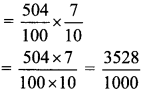= 3.528

Question 4.
Virendra bought 18 bags of rice, each bag weighing 5.250 kg. How much rice did he buy altogether? If the rice costs Rs 42 per kg, how much did he pay for it?
Solution:
Weight of one bag of rice = 5.250 kg
Number of bags of rice = 18
∴ Total Weight = 18 × 5.250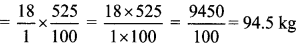Cost of 1 kg of rice = Rs 42
∴ Cost of 94.5 kg of rice = 42 × 94.5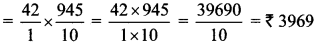∴ Total rice bought by Virendra is 94.5 kg, and the amount paid for it is Rs 3969.

Question 5.
Vedika has 23.5 metres of cloth. She used it to make 5 curtains of equal size. If each curtain required 4 metres 25 cm to make, how much cloth is left over?
Solution:
We know, that 1 m = 100 cm
Cloth required to make 1 curtain = 4 m 25 cm
= 4 m + $$\frac { 25 }{ 100 }$$ m
= 4 m + 0.25 m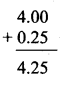= 4.25 m
∴ Cloth required to make 5 curtains = 5 × 4.25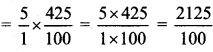= 21.25 m
Cloth remaining with Vedika = Total cloth with Vedika – Cloth used
= 23.5 m – 21.25 m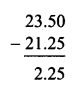= 2.25 m
∴ The length of cloth remaining with Vedika is 2.25 m.

Scroll to Top